# Selina Concise Mathematics Class 9 ICSE Solutions Mid-point and Its Converse [ Including Intercept Theorem]

## Selina Concise Mathematics Class 9 ICSE Solutions Mid-point and Its Converse [ Including Intercept Theorem]

APlusTopper.com provides step by step solutions for Selina Concise Mathematics Class 9 ICSE Solutions Chapter 12 Mid-point and Its Converse [ Including Intercept Theorem]. You can download the Selina Concise Mathematics ICSE Solutions for Class 9 with Free PDF download option. Selina Publishers Concise Mathematics for Class 9 ICSE Solutions all questions are solved and explained by expert mathematic teachers as per ICSE board guidelines.

Selina ICSE Solutions for Class 9 Maths Chapter 12 Mid-point and Its Converse [ Including Intercept Theorem]

Exercise 12(A)

Solution 1: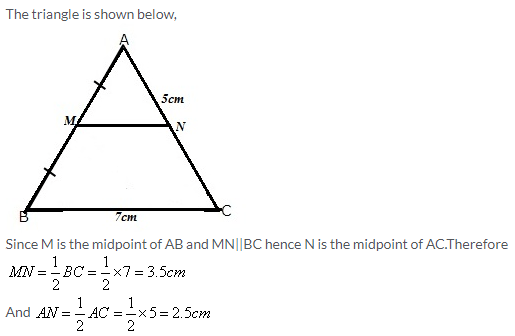Solution 2:Solution 3: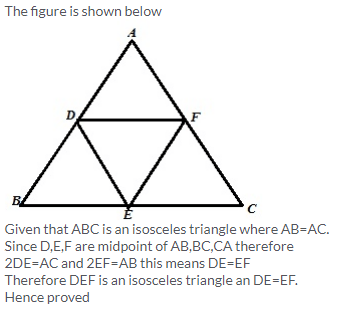Solution 4:Solution 5: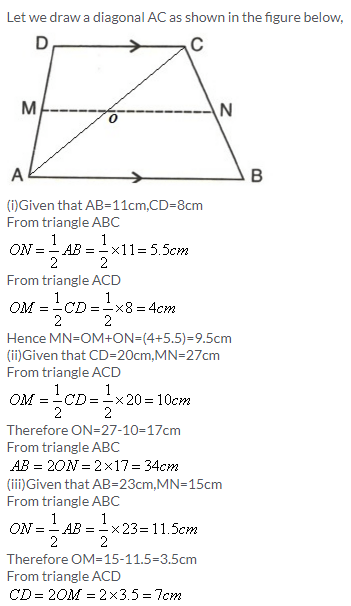Solution 6: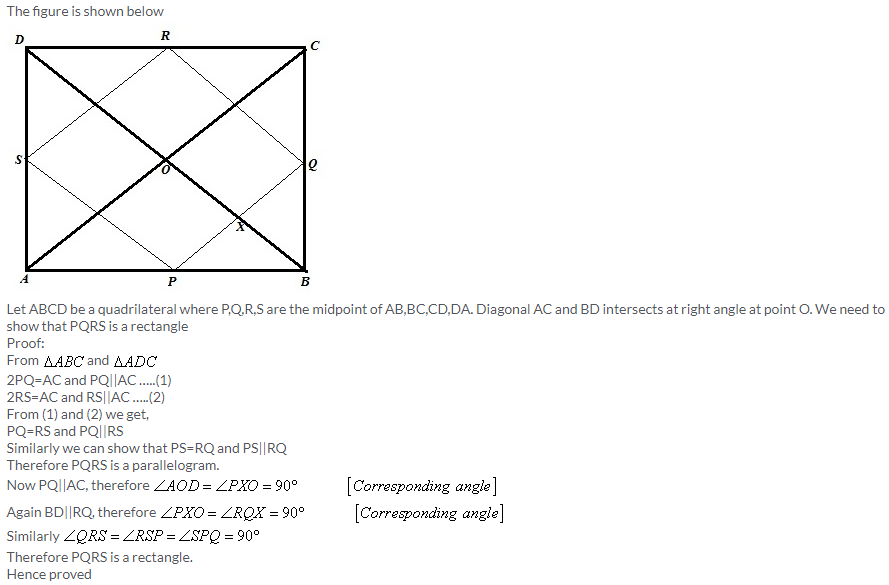Solution 7: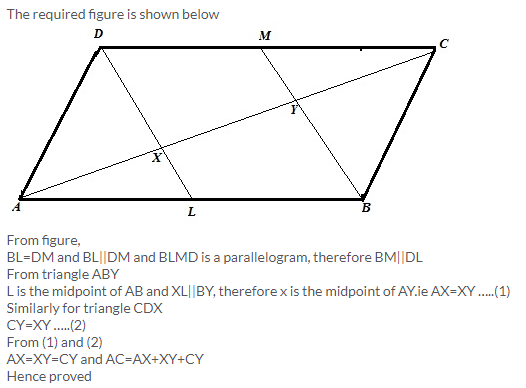Solution 8:Solution 9: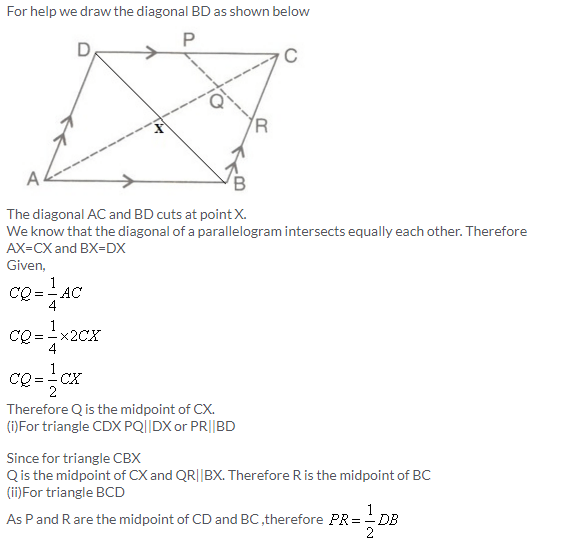Solution 10: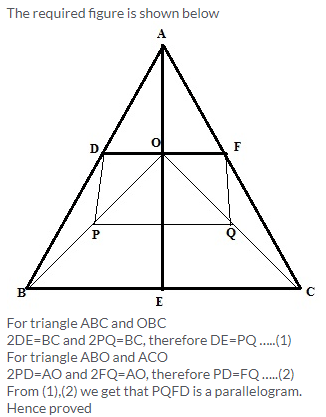Solution 11: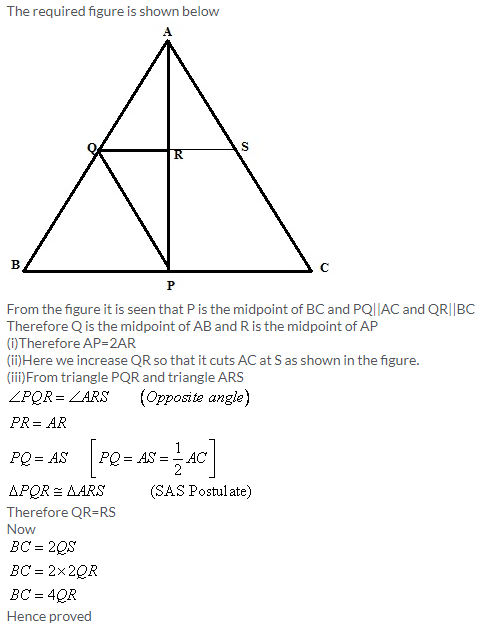Solution 12: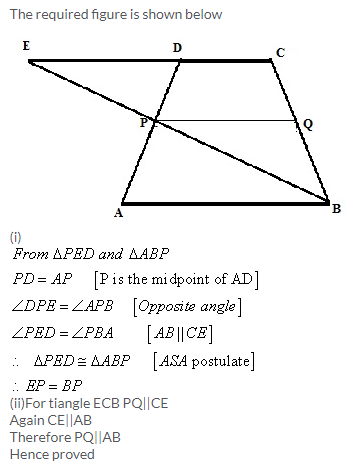Solution 13:Solution 14:Solution 15:Solution 16:Solution 17: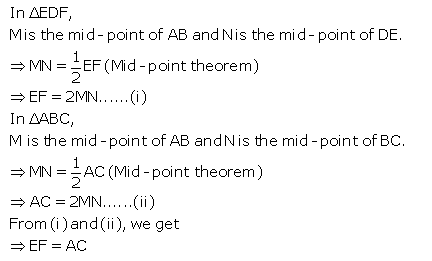Exercise 12(B)

Solution 1: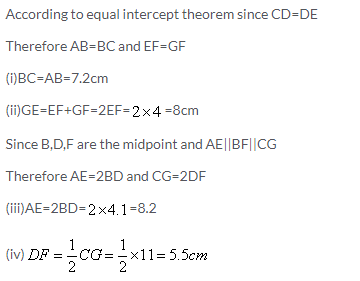Solution 2:Solution 3: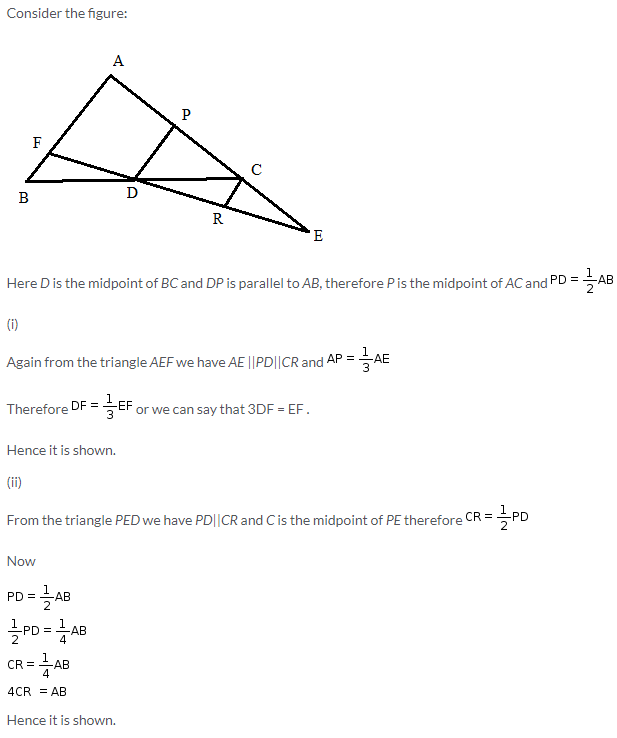Solution 4: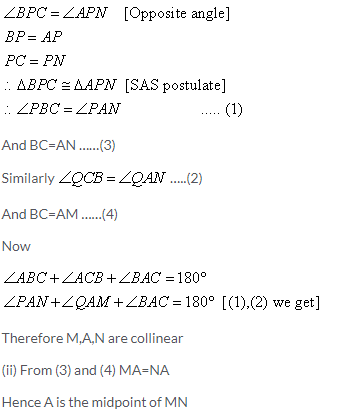Solution 5:Solution 6:Solution 7:Solution 8: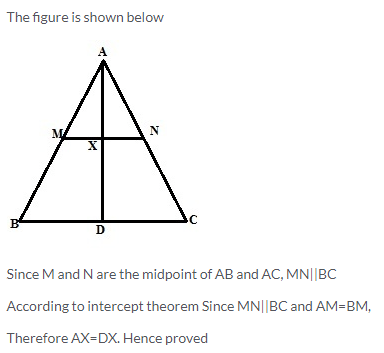Solution 9: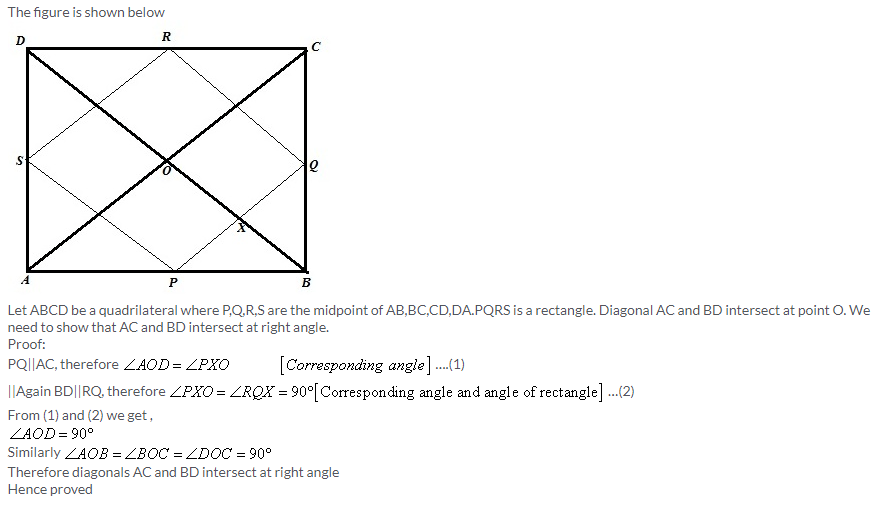Solution 10:Solution 11: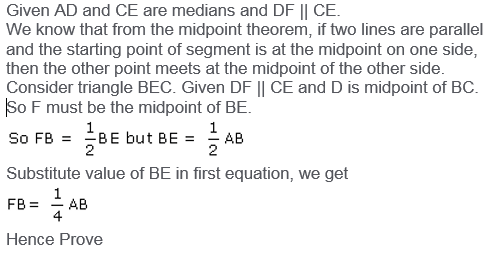Solution 12:
Given ABCD is parallelogram, so AD = BC, AB = CD.
Consider triangle APB, given EC is parallel to AP and E is midpoint of side AB. So by midpoint theorem, C has to be the midpoint of BP.
So BP = 2BC, but BC = AD as ABCD is a parallelogram.
Consider triangle APB, AB || OC as ABCD is a parallelogram. So by midpoint theorem, O has to be the midpoint of AP.
Hence Proved

Solution 13:
Consider trapezium ABCD.
Given E and F are midpoints on sides AD and BC, respectively.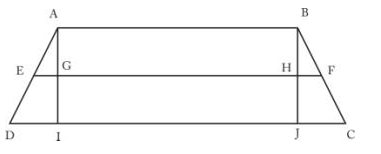Consider LHS,
AB + CD = AB + CJ + JI + ID = AB + 2HF + AB + 2EG
So AB + CD = 2(AB + HF + EG) = 2(EG + GH + HF) = 2EF
AB + CD = 2EF
Hence Proved

Solution 14:
Given Δ ABC
AD is the median. So D is the midpoint of side BC.
Given DE || AB. By the midpoint theorem, E has to be midpoint of AC.
So line joining the vertex and midpoint of the opposite side is always known as median. So BE is also median of Δ ABC.

More Resources for Selina Concise Class 9 ICSE Solutions